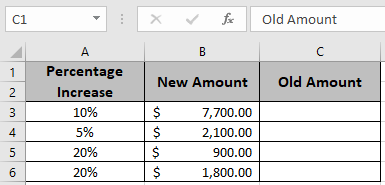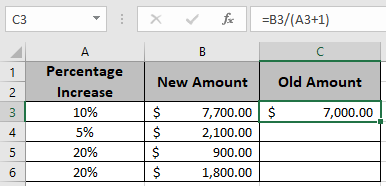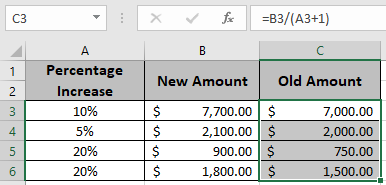# Get Original number In Excel From The Percent Change

In this article, we will learn about how to calculate the old or original number from the percent value and new or updated amount in excel using a formula.
To find the old amount when the new amount and its percentage increase value is given use the formula mentioned in the article.

Generic formula:

Original Amount = New Amount / (1 + Percentage Increase)

Percent increase :

Let’s understand this formula using in the example shown below.

Here we will use the percentage increase value formula to get the original number from percentage. See the example below.Use the Generic Formula in D3 cell

= B3 / ( 1 + C3 )

Explanation : The new amount will be divided by the percentage change plus one.
Here the values to the function are given as cell reference.As you can see in the above snapshot the first original amount before increment is \$ 7,000.00 . Here we have done the reverse calculation of percentage.

Copy the formula in the remaining cells using Ctrl + D shortcut, to get the original amount for the rest of the values in the table.In the above snapshot the mathematical formula used to get the old amount in the excel.

Notes :

1. The function returns #VALUE error if any of the arguments are non numeric.
2. The function returns values for the negative as well as positive values.
3. Use the Increase by percentage formula to get the new or updated amount having percentage increase values.

Hope you understood how to calculate Calculate old or original Amount in Excel. Explore more articles on Mathematical formulation like Increase by percentage and Profit margin percentage in Excel here. Mention your queries in the comment box below. We will help you with it.

Related Articles

Increase by percentage

Calculate Percentage of Total in Excel

Calculate Profit margin percentage

Popular Articles

Edit a dropdown list

If with conditional formatting

If with wildcards

Vlookup by date

Terms and Conditions of use

The applications/code on this site are distributed as is and without warranties or liability. In no event shall the owner of the copyrights, or the authors of the applications/code be liable for any loss of profit, any problems or any damage resulting from the use or evaluation of the applications/code.